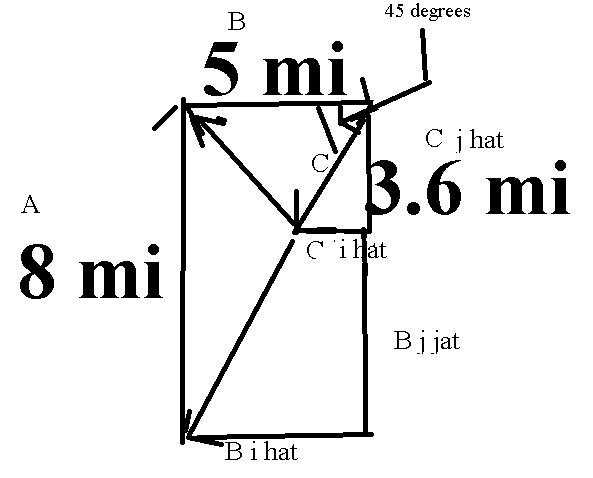# How to solve for vectors components

warnexus

## Homework Statement

A car drives north at 48mi/h for 10 min and then turns east and goes 5.0 mi at 66mi/h . Finally, it goes southwest at 36mi/h for 6.0 min.

Determine the car's displacement.

Assume that the positive x and y axes are directed to the east and north, respectively.
Answers should be in x and y components of the resultant

## Homework Equations

x component aka i hat lies in the x-axis
y component aka j hat lies in the y axis

## The Attempt at a Solution

since the car is going north at that velocity. the magnitude of that vector is 8 mi. When the car goes east, the magnitude is given as 5.0mi. when the car moves southwest, the magnitude is 3.6mi.

Not sure if the graph is right but I thought I get feedback on it.Last edited:

Norfonz
The total displacement is merely the sum of the given vectors. Write each vector in terms of its components. Note that a direction like southwest implies a 45 degree angle between south and west, this should help you write the components.

To find a vector component in a direction, you need to know the angle between the vector and that direction.

Naty1
since the car is going north at that velocity. the magnitude of that vector is 8 mi. When the car goes east, the magnitude is given as 5.0mi. when the car moves southwest, the magnitude is 3.6mi.

correct...

so plot these on graph paper, tail to head [ector arrow point], and connect the final head to the origin...the length is your final magntiude and the angle you measure relative to horizontal records the orientation. ..so for example, go 8 up [vertical], from there 5 down and to the right at 45 degrees 5, then horizontal right from there 3.6...

warnexus
I thought it was 5 horizontal right and 3.6 down left. Thanks for the feedback. i added a picture on what I did with the vector components. I feel super stuck on this problem.